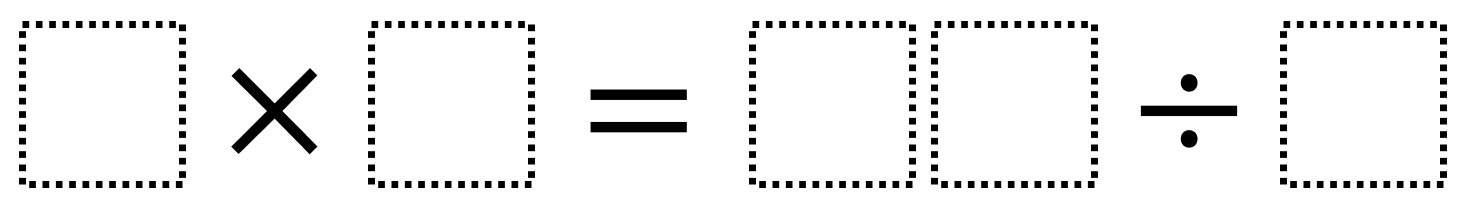# Multiply and Divide Within A Hundred 1

Directions: Using the digits 2 to 9 at most one time each, place a digit in each box to make two correct equations: one where the value is greater than 30 and one less than 30. You may reuse all the digits each equation.### Hint

What digits would be better worse to divide by? What are the greatest values you can make on the division side?

There are many possible answers including 3 x 6 = 72 ÷ 4 (which equals 18 on both sides) as well as 7 x 6 = 84 ÷ 2 (which equals 42 on both sides).

Source: Robert Kaplinsky

## Make The Time

Directions: Using the digits 0 to 9 at most one time each, place a digit …

1.2×8 = 48/3= 16

2.5×5 = 50/2 = 25

•This doesn’t work because you can only use each digit one time.

3.Abigail Selim
2 x 7=28/2=14

•You use the same digit more than once.
Rule is use digit from 2 to 9 only one time…

4.8 x 9=144/2=72

5.4×2 = 16/2 = 8

6.5×5=50/2=25

7.9×4=72/2=36

8.9×5=90/2=45

9.4×4 = 32/2 = 16

10.9×9 = 162/2 = 81

11.8×7 = 112/2 = 56

12.9×2 = 162/9 = 18

13.6×7= 126/3= 42

14.12×8= 192/2= 96

15.10 x 4 = 40 / 5 =8

16.8×3=48/2=24

17.74×2=296/2=148

18.8×8=128/2=64

19.9×8=216/3=72

20.7×7=98/2=49

21.5×1=100/20=5

22.Kimberly Kamborian

You may reuse all the digits each equation? Is the multiplication and division expressions considered two different equations?

So can we do 2 x 3 = 42/7

23.3 x 4 = 72 / 6

24.6 x 4 = 72 / 3

25.Ava & Mrs. Hook

1×6=54/9

They both equal 6.
=)

26.One would be 3 x 9 = 54 / 2 = 27, and another could be 7 x 6 = 84 / 2 =42

27.7×6=84/2

28.3×2=54/9